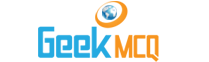# Entry Test MCQ :: Electromagnetic Induction

1.  The practical illustration of the phenomenon of mutual induction is
 A. A.C generator B. D.C dynamo C. induction coil D. transformer

2.  Weber is the unit of
 A. Magnetic field intensity B. magnetic induction C. magnetic flux D. self-inductance

3.  Current produced by moving the loop of wire across a magnetic field is called
 A. A.C current B. D.C current C. induced current D. mean square current

4.  emf induced in a circuit according to Faradays law depends on the
 A. maximum magnetic flux B. rate of change of magnetic flux C. change in magnetic flux D. initial magnetic flux

5.  cmf generated by A.C dynamo depends upon
 A. number of turns in the coil B. magnetic field strength C. frequency of rotation D. all of above

6.  An alternating current or voltage
 A. fluctuates off and on B. varies in magnitude alone C. changes its direction again and again D. changes its magnitude continuously and reverses its direction of flow after regularly recurring intervals.

7.  A dynamo converts
 A. mechanical energy into electrical energy B. electrical energy into mechanical energy C. magnetic energy into mechanical energy D. magnetic energy into electrical energy

8.  Which one of the following functions like a motor?
 A. galvanometer B. ammeter C. voltmeter D. all of above

9.  A.C cannot be used for
 A. producing heat B. producing light C. magnetizing and electroplating D. all the above

10.  Which of the following works on torque on the current carrying conductor placed in magnetic field.
 A. galvanometer B. ammeter C. voltmeter D. all of the above